# RD Sharma Solutions -Ex-20.2, Surface Area And Volume Of Right Circular Cone, Class 9, Maths Notes | Study RD Sharma Solutions for Class 9 Mathematics - Class 9

## Class 9: RD Sharma Solutions -Ex-20.2, Surface Area And Volume Of Right Circular Cone, Class 9, Maths Notes | Study RD Sharma Solutions for Class 9 Mathematics - Class 9

The document RD Sharma Solutions -Ex-20.2, Surface Area And Volume Of Right Circular Cone, Class 9, Maths Notes | Study RD Sharma Solutions for Class 9 Mathematics - Class 9 is a part of the Class 9 Course RD Sharma Solutions for Class 9 Mathematics.
All you need of Class 9 at this link: Class 9

Q1.Find the volume of the right circular cone with the following dimensions:

(a)Radius is 6cm and the height of the cone is 7cm

(b)Radius is 3.5cm and height is 12cm

(c)Slant height is 21cm and height is 28cm

Solution:

(a) It is given that

Radius of the cone(r) = 6cm

Height of the cone(h) = 7cm

Volume of  a right circular cone

=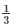πr2h

=∗3.14∗62∗7  = 264 cm3

(b) It is given that:

Radius of the cone(r) = 3.5 cm

Height of the cone(h) = 12cm

Volume of  a right circular cone

=πr2h

=∗3.14∗3.52∗12

= 154 cm3

(c) It is given that:

Height of the cone(h) = 28 cm

Slant height of the cone(l) = 21 cm

As we know that,

l2 = r2+h2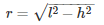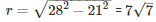Volume of a right circular cone:

=πr2h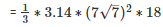= 7546cm3

Q2.Find the volume of a conical tank with the following dimensions in liters:

(a)Radius is 7cm and the slant height of the cone is 25cm

(b)Slant height is 12cm and height is 13cm

Solution:

(a) It is given that

Radius of the cone(r) = 7 cm

Slant height of the cone (l) = 25 cm

As we know that,

l2 = r2+h2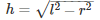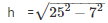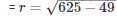= 24 cm

Volume of a right circular cone

=πr2h

=∗3.14∗72∗24

= 1232cm = 1.232 litres   [1 cm3 = 0.01l]

(c) It is given that:

Height of the cone(h) = 12 cm

Slant height of the cone(l) = 13 cm

As we know that,

l2 = r2+h2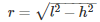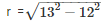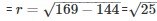= 5cm

Volume of a right circular cone:

=πr2h

=∗3.14∗52∗10  = 314.85cm = 0.307litres   [1 cm3 = 0.01l]

Q3. Two cones have their heights in the ratio 1:3 and the radii of their bases in the ratio 3:1.Find the ratio of their volumes.

Solution:

Let ratio of the height of the cone be hx

height of the 1st cone =  hx

height of the of the  2nd cone = 3hx

Let the ratio of the radius of the of the cone =  rx

radius  of the   1st cone = 3 rx

radius  of the  2nd cone = rx

The ratio of the volume =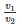Where v= volume of 1st cone

v2 = volume of 2nd cone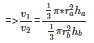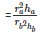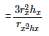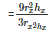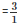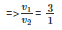Q4. The radius and the height of a right circular cone are in the ratio 5:12. If its volume is 314 cubic meter, find the slant height and the radius. (Use π = 3.14).

Solution:

Let us assume the ratio to be y

Height (h) = 12y

We know that

l2 = r2+h2

= 5y2+12y2

= 25y2+144y2

= 1692  = 13y

Now it is given that volume = 314m3πr2h = 314m3∗3.14∗25y2∗12y = 314m3

⇒ y3 = 1

⇒ y = 1

Therefore ,

Slant height(l) = 13y = 13m

Q5. The radius and height of a right circular cone are in the ratio 5 : 12 and its volume is 2512 cubic cm. Find the slant height and radius of the cone. (Use π = 3.14).

Solution:

Let the ratio be y

The radius of the cone(r) = 5y

Height of the cone = 12y

Now we know,

Slant height(l) =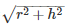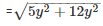= 13y

Now the volume of the cone is given 2512cm3πr2h = 2512∗3.14∗5y2∗12y = 2512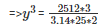⇒ y = 2

Therefore,

Slant height(l) = 13y = 13*2 = 26cm

Radius of cone = 5y = 5*2 = 10cm

Q6. The ratio of volumes of two cones is 4 : 5 and the ratio of the radii of their bases is 2 : 3. Find the ratio of their vertical heights.

Solution:

Let the ratio of the radius be x

Radius of 1st cone = 2x

Radius of 2nd cone = 3x

Let the ratio of the volume be y

Volume of 1st cone = 4y

Volume of 2nd cone = 5y

=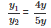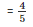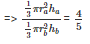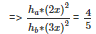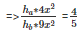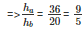Therefore the heights are in the ratio of 9:5.

Q7. A cylinder and a cone have equal radii of their bases and equal heights. Show that their volumes are in the ratio 3:1.

Solution:

It’s given that

A cylinder and a cone are having equal radii of their bases and heights

Let the radius of the cone = radius of the cylinder = r

Height of the cone = height of the cylinder = h

Let the volume of cone = vx

Volume of cylinder = vy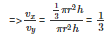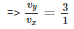Therefore the ratio of their volumes is 3:1.

Q8. If the radius of the base of a cone is halved, keeping the height same, what is the ratio of the volume of the reduced cone to that of the original cone?

Solution:

Let the radius of the cone be rxandheightbehx

Then the volume of cone vx =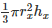Now,

Radius of the reduced cone =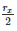Therefore volume of reduced cone vy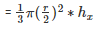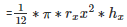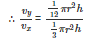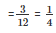Therefore the ratio between the volumes of the reduced and the original cone is 1:4.

Q9. A heap of wheat is in the form of a cone of diameter 9 m and height 3.5 m. Find its volume. How much is canvas cloth required to just cover the heap?  (Use π = 3.14).

Solution:

It is given that

Diameter of heap(d) = 9m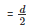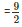= 4.5m

Height of the heap(h) = 3.5m

Therefore, Volume of the heap =πr2h

=∗3.14∗4.52∗3.5

= 74.18m3

Now,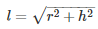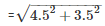= 5.70m

Area to be covered by the cloth = Curved surface area of the heap

= πrl  = 3.14*4.5*5.70 = 80.54 m3

Q10. Find the weight of a solid cone whose base is of diameter 14 cm and vertical height 51 cm, supposing the material of which it is made weighs 10 grams per cubic cm.

Solution:

It is given that :

Diameter(d) = 14cm

Height of the cone(h) = 51cm

Radius of the cone(r) =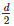=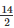= 7cm

Therefore, Volume of  cone(V) =πr2h =

=*3.14*7*5*51 = 2618 cm3

Now it is given that 1 cm3 material weighs 10gm.

Therefore, 2618cm3

weighs = 2618*10 = 26180 grams.

Q11. A right angled triangle of which the sides containing the right angle are 6.3 cm and 10 cm in length, is made to turn round on the longer side. Find the volume of the solid, thus generated. Also, find its curved surface area.

Solution:

It is given that

Height of the cone(h) = 10cm

We know that

Slant height(l) =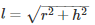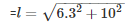= 11.819cm

Therefore Volume of cone(v) =πr2h

=∗3.14∗6.32∗10  = 415.8cm3

Curved surface area of cone = πrl

= 3.14*6.3*11.819 = 234.01cm2

Q12 Find the volume of the largest right circular cone that can be fitted in a cube whose edge is 14 cm.

Solution:

Radius of the base of the largest cone =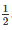*edge of the cube

=*14 = 7cm

Height of the cone = Edge of the cube = 14cm

Therefore, Volume of cone(v) =πr2h

=∗3.14∗72∗14  = 718.66 cm3

Q13 The volume of a right circular cone is 9856 cm3. If the diameter of the base is 28 cm. Find:

(a)Height of the cone

(b)Slant height of the cone

(c)Curved surface area of the cone

Solution:

(a) It is given that diameter of the cone(d) = 28cm

Radius of the cone(r) ==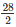= 14cm

Height of the cone = ?

Now,

Volume of the cone(v) =πr2h = 9856 cm3∗3.14∗142∗h = 9856

⇒ h =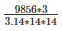= 48cm

Therefore the height of the cone is 48cm

(b) It is given that

Radius of the cone(r) = 14cm

Height of the cone = 48cm

Slant height (l) = ?

Now we know that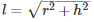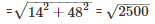= 50cm

Therefore the slant height of the cone is 50cm.

(c) Radius of the cone(r) = 14cm

Slant height of the cone(l) = 50cm

Curved surface area(C.S.A) = ?

Curved surface area of a cone (C.S.A) =  πrl

= 3.14*14*50 = 2200 cm2

Therefore curved surface of the cone is 2200 cm2

Q14. A conical pit of top diameter 3.5m is 12m deep. What is its capacity in kilolitres?

Solution:

It is given that :

Diameter of the conical pit(d) = 3.5m

Height of the conical pit(h) = 12m

Radius of the conical pit(r) = ?

Volume of the conical pit(v) = ?

Radius of the conical pit(r) =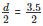= 1.75m

Volume of the cone(v) =πr2h

=∗3.14∗1.752∗12  = 38.5m3

Capacity of the pit = (38.5*1 )kilolitres = 38.5 kilolitres

Q15. Monica has a piece of Canvas whose area is 551m2. She uses it to have a conical tent made, with a base radius of 7m. Assuming that all the stitching margins and wastage incurred while cutting amounts to approximately 1 m2. Find the volume of the tent that can be made with it.

Solution:

It is given that:

Area of the canvas = 551m2

Area that is wasted = 1m2

Radius of tent = 7m  , Volume of tent(v) = ?

Therefore the Area of available for making the tent = (551-1) = 550 m2

Surface area of tent = 550 m2

⇒ πrl   = 550

⇒ l =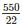= 25m

Slant height(l) = 25m

We know that,

l2 = r2+h2

252 =  72+h2

⇒ 625-49 = h2

⇒ 576 = h2

h = 24m

Height of the tent is 24m.

Now, volume of cone =πr2h

=∗3.14∗72∗24  = 1232 m3

Therefore the volume of the conical tent is 1232 m3..

The document RD Sharma Solutions -Ex-20.2, Surface Area And Volume Of Right Circular Cone, Class 9, Maths Notes | Study RD Sharma Solutions for Class 9 Mathematics - Class 9 is a part of the Class 9 Course RD Sharma Solutions for Class 9 Mathematics.
All you need of Class 9 at this link: Class 9Use Code STAYHOME200 and get INR 200 additional OFF

## RD Sharma Solutions for Class 9 Mathematics

91 docs

Track your progress, build streaks, highlight & save important lessons and more!

,

,

,

,

,

,

,

,

,

,

,

,

,

,

,

,

,

,

,

,

,

,

,

,

,

,

,

,

,

,

;